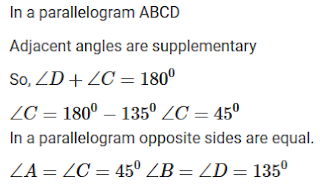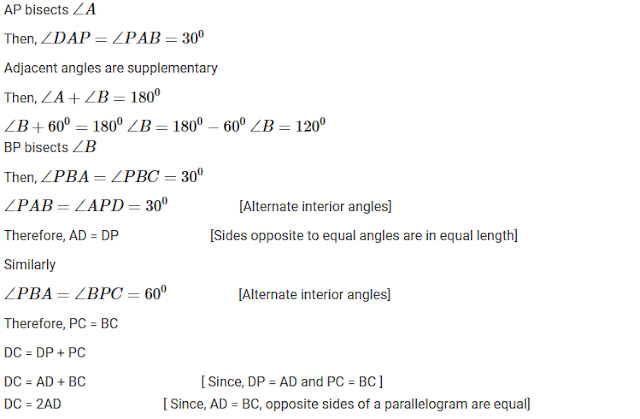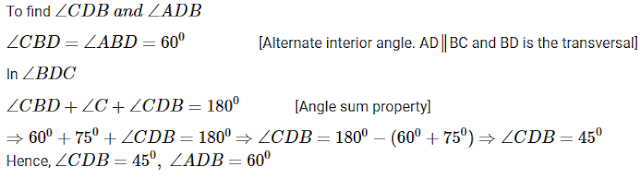#### Chapter 14 Quadrilaterals R.D. Sharma Solutions for Class 9th Exercise 14.2

Exercise 14.2

1. Two opposite angles of a parallelogram are (3x − 2)° and (50 − x)°. Find the measure of each angle of the parallelogram.

Solution2. If an angle of a parallelogram is two-third of its adjacent angle, find the angles of the parallelogram.

Solution3. Find the measure of all the angles of a parallelogram, if one angle is 24° less than twice the smallest angle.

Solution4. The perimeter of a parallelogram is 22 cm. If the longer side measures 6.5 cm what is the measure of the shorter side?

Solution5. In a parallelogram ABCD, ∠D = 135°, determine the measures of ∠A and ∠B.

Solution6. ABCD is a parallelogram in which ∠A = 70°. Compute ∠B, ∠C and ∠D.

Solution7. In Fig. 14.34, ABCD is a parallelogram in which ∠A = 60°. If the bisectors of ∠A and ∠B meet at P, prove that AD = DP, PC = BC and DC = 2AD.Solution8.  In Fig. 14.35, ABCD is a parallelogram in which ∠DAB = 75° and ∠DBC = 60°. Compute ∠CDB and ∠ADB.Solution9. In figure 14.36, ABCD is a parallelogram and E is the mid-point of side BC. If DE and AB when produced meet at F, prove that AF = 2AB.Solution10. Which of the following statements are true (T) and which are false (F)?
(i) In a parallelogram, the diagonals are equal.
(ii) In a parallelogram, the diagonals bisect each other.
(iii) In a parallelogram, the diagonals intersect each other at right angles.
(iv) In any quadrilateral, if a pair of opposite sides is equal, it is a parallelogram.
(v) If all the angles of a quadrilateral are equal, it is a parallelogram.
(vi) If three sides of a quadrilateral are equal, it is a parallelogram.
(vii) If three angles of a quadrilateral are equal, it is a parallelogram.
(viii) If all the sides of a quadrilateral are equal, it is a parallelogram.

Solution

(i) False
(ii) True
(iii) False
(iv) False
(v) True
(vi) False
(vii) False
(viii) True